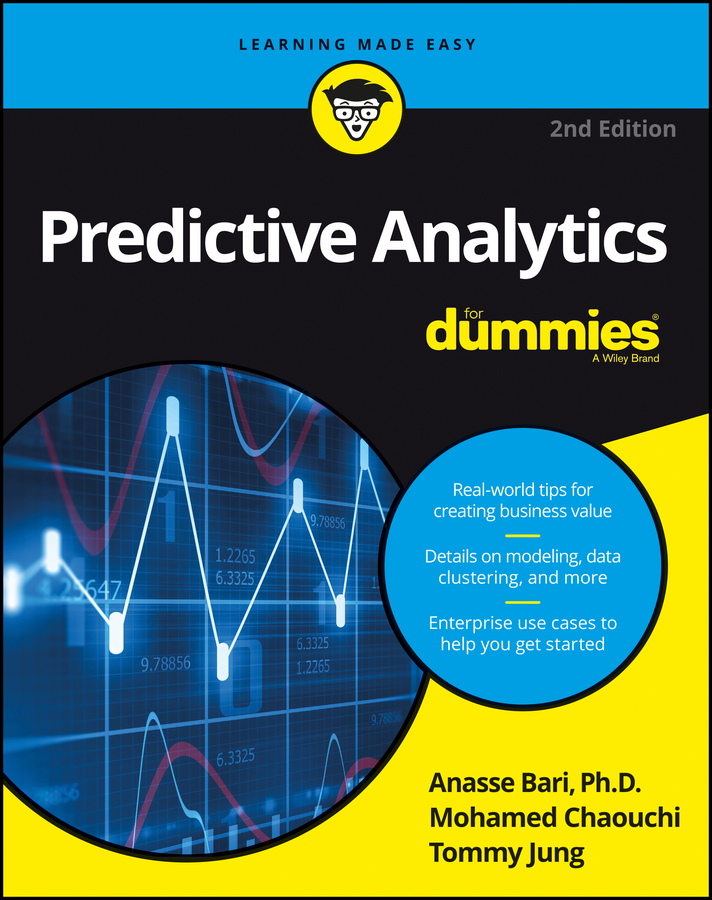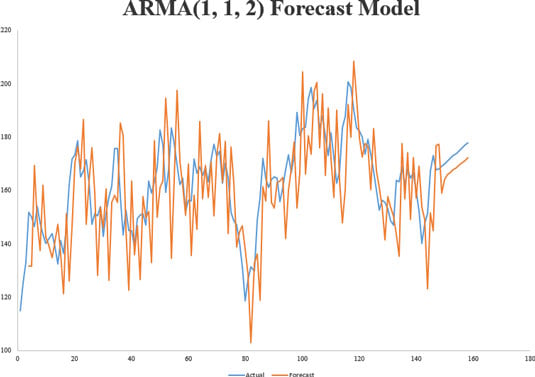##### Predictive Analytics For DummiesSimilar to how multivariate analysis is the analysis of relationships between multiple variables, univariate analysis is a quantitative analysis of only one variable. When you model univariate time series, you are modeling time series changes that represent changes in a single variable over time.

Autoregressive Moving Average (ARMA) is a class of forecasting methods that you can use to predict future values from current and historical data. As its name implies, the family of ARMA models combines autoregression techniques (analyses that assume that previous observations are good predictors for future values and perform an autoregression analysis to forecast for those future values) and moving average techniques — models that measure the level of the constant time series and then update the forecast model if any changes are detected.

Here is an example of an equation for the ARMA model:

ARMA(p, q)
yt = a1yt1 + a2yt2 + . . . + apyt p + b0 + etb1et 1b2et‐]2 + . . . – bqet q

In this equation

• yt equals the actual value of the time series at time t.

• yt 1 equals the actual value of the time series at time t – 1.

• yt yt1 equals the net change in the value of time series between time t and time t – 1 — the change in value of the time series over one interval of time, in other words.

• et‐]1 equals the error term at time t – 1 (a quantification of the error processes in the model at time t – 1).

• a1 equals the autoregressive parameter for yt 1.

• b1 equals the moving average parameter for et 1.

• et, et 1, et 2, . . . , and et q are uncorrelated.

Autoregression assumes that the previous p observations in the time series provide a good estimate of future observations. The moving average part of the model allows the model to update the forecasts if the level of a constant time series changes. If you’re looking for a simple model or a model that will work for only a small dataset, then the ARMA model is not a good fit for your needs. An alternative in this case might be to just stick with simple linear regression.

In order to use the ARMA model for reliable results, you need to have at least 50 observations and a trained analyst who can fit and interpret the model for you.

The figure shows a chart of the ARMA model that corresponds to this equation:

yt = 0.91yt 1 + 11.6 – 1.412yt 1 + 0.987yt 2

In the figure, you can see that the model forecast data and the actual data are a very close fit. This means that the equation that formulated above is a good representation of the time series it models.